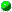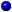SYLLABUS  Previous: 1.6 Computer quiz  Up: 1 INTRODUCTION  Next: 1.8 Further reading and

1.7 Exercises1.00 E-learning.1.01 Model portfolio.1.02 Cost averaging.1.03 Performance of an investment.1.04 Investment risk.1.05 Volatility measurements.1.06 Stock valuation.1.07 Zero rate of coupon bearing bonds.1.08 UWMA for the volatility of interest rates.1.09 EWMA for the drift of shares.1.10 GARCH variance targeting.1.11 Forecasting volatility.

IMPORTANT: All these problems can be edited and submitted for correction by selecting WORK:assignments on the course main page. They generally open with a template that provides useful advice and guidance to derive the solution. The color coding corresponds to the expected level of difficulty:Easy.
The problem is a direct application of the theory in a situation of practical interest: solutions typically combine text without formulas, ASCII plots and parameters of the VMARKET applet without any programming.Moderate. generally be solved with a short analytical derivation, a discussion of the result, a plot parametric dependencies.Difficult. The problem is an extension of the material discussed in the syllabus and requires a good command of the mathematical language, which can be expected for university graduates from quantitative fields.
SYLLABUS  Previous: 1.6 Computer quiz  Up: 1 INTRODUCTION  Next: 1.8 Further reading and